• ## 坐标系变换

万次阅读 多人点赞 2017-04-29 14:23:44
坐标系变换坐标系变换 不考虑平移 加入平移 齐次坐标系与平移 追溯过程 矩阵变换坐标系变换 or 位置变换 移动的坐标系 Think an invertible matrix as a coordinate. 坐标系变换，例如，现在有一个点P，在A坐标系中...
坐标系变换

坐标系变换
不考虑平移
加入平移
齐次坐标系与平移

追溯过程
矩阵变换坐标系变换 or 位置变换
总结

Think an invertible matrix as a coordinate.

坐标系变换，例如，现在有一个点P，在A坐标系中的坐标为(x,y)$(x,y)$，如何求出点P在其他坐标系下的坐标呢？比如，我们想知道P点在B坐标系中的坐标？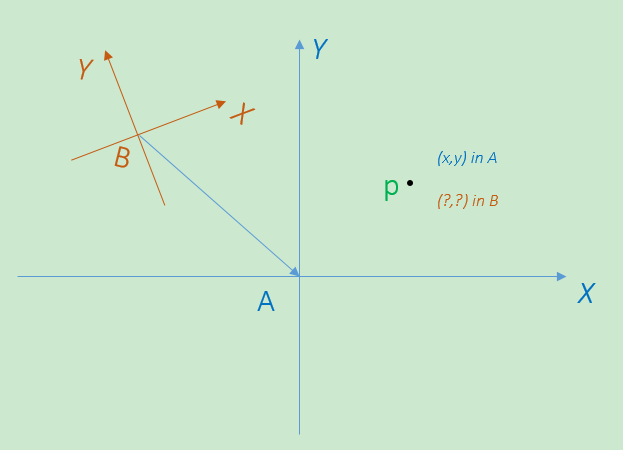做个类比，如果两个讲不同母语的人想要交流，怎么办？这时，如果有一种世界通用语言就好了，例如汉语。这样，当说英语的人向说法语的人问候“你好”的时候，他知道要查字典先把英文 “hello”转换成中文“你好”，然后，说法语的人就可以将“你好”转换成法文“Bonjour”。

这里有两个过程：

hello →$\rightarrow$ 你好
你好→$\rightarrow$ Bonjour

这两个过程中有一个关键点：

你好 —汉语

是的，世界通用语言，我们的汉语，就是key point， 她在不同语系中充当了桥梁的角色。

回到坐标系的话题，使用不同的坐标系就像使用不同的语言，各说各话，相互之间是无法被理解的。如果想要被理解，那就的使用大家都认同的方式交流。这时候，如果引入一个参考坐标系，世界就和平了：

我们可以将P点的坐标先转化为一个参考坐标，然后再从参考坐标转换到B坐标系中的坐标。

A →$\rightarrow$ Global
Global →$\rightarrow$ B

现在，我们的问题变成了求某个坐标系X与参考坐标系Global之间的转换方法：

X ↔$\leftrightarrow$ Global

不考虑平移

如果考虑不同的坐标系共原点，就像下面这样：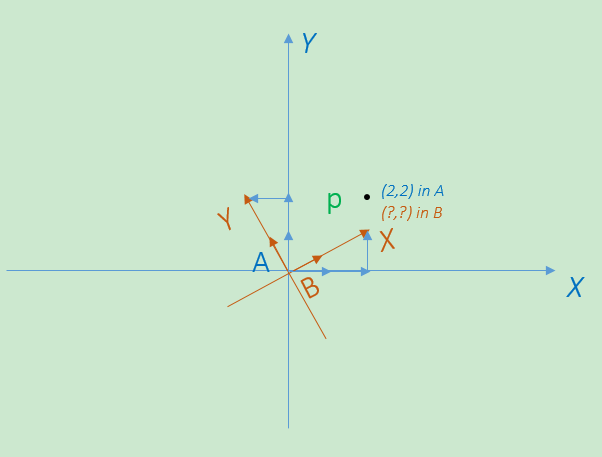选择坐标系A作为参考坐标系，如何表述：

B ↔$\leftrightarrow$ A

答案是：矩阵变换

矩阵变换就像词典翻译一样，将一个坐标系下的点转换成另一个坐标系中的点。

矩阵与线性变换中提到了在同一个坐标系中矩阵变换的作用，例如，将一个向量进行旋转。现在，矩阵变换被不可思议的用作了坐标系变换的工具。变换还是同样的变换，只是站在了不用的角度看待问题：

矩阵变换之于同一个坐标系，可以理解为坐标系不变，点的位置改变。
矩阵变换之于不同坐标系，可以理解为点的绝对位置不变，坐标系改变。

其实在矩阵与线性变换拓展一节中，我提到了一种转换关系，这里，我给出上图的对应表述：

(1)   [x′y′]$\begin{bmatrix}x'\\y'\end{bmatrix}$ = B[xy]$B\begin{bmatrix}x\\y\end{bmatrix}$ ⇒$\Rightarrow$ [xy]$\begin{bmatrix}x\\y\end{bmatrix}$ = B−1[x′y′]$B^{-1}\begin{bmatrix}x'\\y'\end{bmatrix}$ ，B$B$=[b1→b2→]$\begin{bmatrix}\vec{b_1}&\vec{b_2}\end{bmatrix}$， 且b1→，b2→$\vec{b_1} ，\vec{b_2}$ 是坐标系B的基向量

其中，矩阵B$B$的各个列向量分别对应B坐标系的各个基向量，[xy]$\begin{bmatrix}x\\y\end{bmatrix}$是向量OP→$\vec{OP}$或者说点P在B坐标系的表示， [x′y′]$\begin{bmatrix}x'\\y'\end{bmatrix}$则是向量OP→$\vec{OP}$或者点P在A坐标系中的表示。求出矩阵B$B$，我们就有了翻译用的字典。

这一切，在于矩阵B$B$的各个列向量，在于B坐标系的基向量。

(1)  的转换关系之所以成立，是因为矩阵B$B$的各个列向量是B坐标系的基向量，他们都是坐标系A中的向量。更明确的说，矩阵B$B$的列向量由坐标系A的基向量通过一个线性变换得到，这个线性变换完全可以用矩阵B$B$表示，详见矩阵与线性变换。

上图对应的矩阵B的两个列向量分别为b1→$\vec{b_1}$=[25√/55√/5]$\begin{bmatrix}2\sqrt{5}/5\\ \sqrt{5}/5\end{bmatrix}$， b2→$\vec{b_2}$=[−5√/525√/5]$\begin{bmatrix}-\sqrt{5}/5\\ 2\sqrt{5}/5\end{bmatrix}$。

其实A、B可以是任何坐标系，式(1)仍然成立，只要满足：

坐标系A和坐标系B原点均为(0,0)
矩阵B$B$的各个列向量分别对应B坐标系的各个基向量
矩阵B$B$的各个列向量都是坐标系A中的向量

坐标系B的基向量是坐标系A的基向量的一个线性变换 ，这个线性变换可以用矩阵B$B$表示(T(x⃗ )=Bx⃗ $T(\vec{x}) = B\vec{x}$)，确定坐标系B的基向量在A坐标系中的表示的过程叫做B坐标系的定位(landed)。将B坐标系的基向量定位在A坐标系中确定了一种关系，我们暂且把这种关系称为追溯关系，把A坐标系称为B坐标系的上游坐标系。反过来，如果把坐标系A的基向量定位在B坐标系中，那么B坐标系就成了A坐标系的上游坐标系。

下面，用图说话：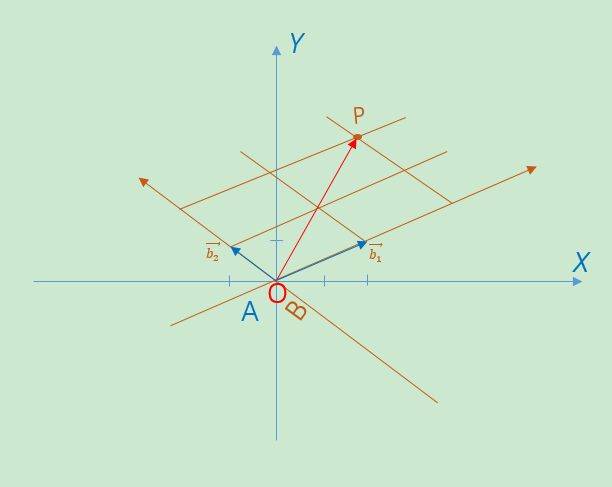以图中的两个向量b1→,b2→$\vec{b_1} ,\vec{b_2}$为基确定一个坐标系B，显然，在B坐标系中b1B→=,b2B→=$\vec{b_{1B}}=\begin{bmatrix}1\\0\end{bmatrix} ,\vec{b_{2B}}=\begin{bmatrix}0\\1\end{bmatrix}$， 接下来，将b1→,b2→$\vec{b_1} ,\vec{b_2}$定位到A坐标中，得到b1A→=,b2A→=[−11]$\vec{b_{1A}}=\begin{bmatrix}2\\1\end{bmatrix} ,\vec{b_{2A}}=\begin{bmatrix}-1\\1\end{bmatrix}$。

∵$\because$   OP→$\vec{OP}$ = 2b1→$\vec{b_1}$+2b2→$\vec{b_2}$

∴$\therefore$  OP→$\vec{OP}$在B坐标系中的表示为$\begin{bmatrix}2\\2\end{bmatrix}$，现在，将OP→$\vec{OP}$用A坐标系描叙：

OP→$\vec{OP}$ = 2b1→$\vec{b_1}$+2b2→$\vec{b_2}$ = 2b1A→+2b2A→$2\vec{b_{1A}}+2\vec{b_{2A}}$ = [b1A→b2A→]$\begin{bmatrix}\vec{b_{1A}}&\vec{b_{2A}}\end{bmatrix}$$\begin{bmatrix}2\\2\end{bmatrix}$ = $\begin{bmatrix}2\\4\end{bmatrix}$

现在，令矩阵B$B$ = [b1A→b2A→]$\begin{bmatrix}\vec{b_{1A}}&\vec{b_{2A}}\end{bmatrix}$，P点是用B坐标系表示的任意一点(x,y)$(x,y)$。

于是OP→$\vec{OP}$在A坐标系中的表示[x′y′]$\begin{bmatrix}x'\\y'\end{bmatrix}$ = B[xy]$\begin{bmatrix}x\\y\end{bmatrix}$ ，显然，B$B$是可逆的，于是就有了(1)式的结论。

虽然这里的讨论是基于二维的，但是，不难想象，这里得出的结论可以扩展到任意维度。

用一句话来阐述这个结论：

将B坐标系的基向量定位到A坐标系，然后将定位之后的基向量作为矩阵B$B$的列向量，用矩阵B$B$对B坐标系中的点P的坐标进行矩阵变换，将得到点P在A坐标系中的坐标。这个过程，就是从坐标系B到坐标系A的一个追溯过程。

加入平移

平移好像没什么好说的，不过就是在前面讨论的基础上引入一个偏移。

下面用图示说明：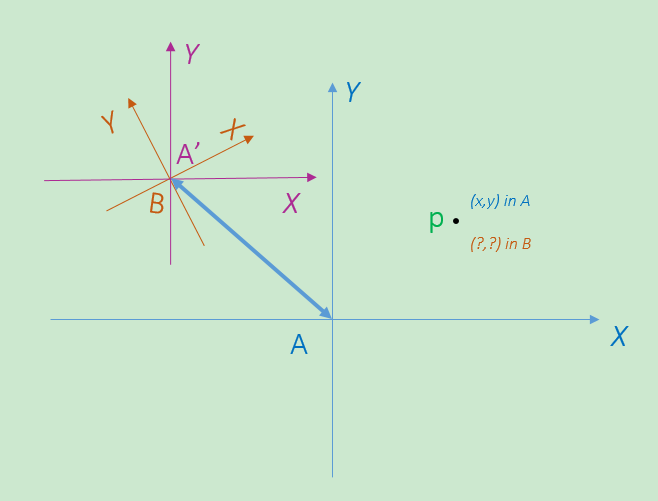上图引入了一个新的坐标系，取名为A’。将这个坐标系取名为A’是有原因的，因为他基本就是坐标系A的一个替身，他们之间除了坐标原点发生偏移之外，其他特征完全一致。

有了前面的基础，我们知道，将坐标系B中的坐标转换为坐标系A’中的坐标其实就是一个从B到A’的追溯过程。只要将坐标系B的基定位到A’坐标系中，以定位之后的基向量为列组成矩阵B$B$，然后对坐标系中的坐标进行B$B$矩阵转换就能得到其在A’坐标系中的坐标。反过来，求出矩阵B$B$的逆，我们就能将A’坐标系中的坐标转化为B坐标系中的坐标。

也就是说，利用前面讨论的结论，我们能完成：

B↔A′$B \leftrightarrow A'$

[x′y′]$\begin{bmatrix}x'\\y'\end{bmatrix}$ = B[xy]$B\begin{bmatrix}x\\y\end{bmatrix}$ ⇒$\Rightarrow$ [xy]$\begin{bmatrix}x\\y\end{bmatrix}$ = B−1[x′y′]$B^{-1}\begin{bmatrix}x'\\y'\end{bmatrix}$

剩下的问题就是：

A′↔A$A' \leftrightarrow A$

这个问题就是一个加减偏移量的问题，所以看图就一目了然了。

齐次坐标系与平移

引入齐次坐标的好处之一是可以使用矩阵表达平移：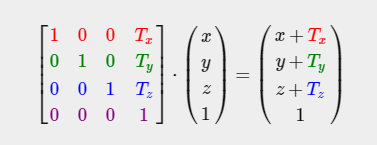所以A′↔A$A' \leftrightarrow A$之间的变换可以用这么一个矩阵变换来完成。

追溯过程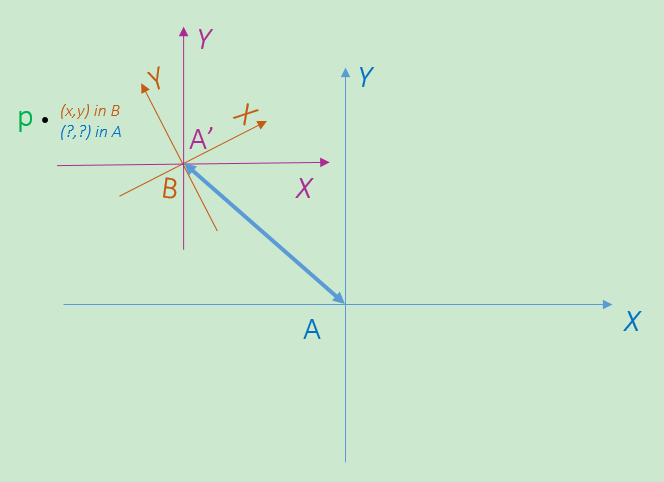将坐标系B的基向量定位到坐标系A’中，得到向量b1A′→,b2A′→$\vec{b_{1A'}} ,\vec{b_{2A'}}$，所以从坐标系B追溯到坐标系A’的矩阵B$B$ = [b1A′→b2A′→]$\begin{bmatrix}\vec{b_{1A'}}&\vec{b_{2A'}}\end{bmatrix}$。A′→A$A' \rightarrow A$的平移矩阵T$T$为 ⎡⎣⎢100010TxTy1⎤⎦⎥$\begin{bmatrix}1&0&T_x\\0&1&T_y\\0&0&1\end{bmatrix}$。

故，将坐标系B中的点p(xB,yB)$p(x_B,y_B)$追溯到坐标系A中可以用下面的矩阵变换表示：

1). 将坐标变换到坐标系A’：

[xA′yA′]$\begin{bmatrix}x_{A'}\\y_{A'}\end{bmatrix}$=B$B$[xByB]$\begin{bmatrix}x_B\\y_B\end{bmatrix}$

2). 将坐标变换到坐标系A中：

[xAyA]$\begin{bmatrix}x_{A}\\y_{A}\end{bmatrix}$=T$T$[xA′yA′]$\begin{bmatrix}x_{A'}\\y_{A'}\end{bmatrix}$

综合起来就是：

[xAyA]$\begin{bmatrix}x_{A}\\y_{A}\end{bmatrix}$=T$T$B$B$[xByB]$\begin{bmatrix}x_B\\y_B\end{bmatrix}$

反过来，A→A′$A\rightarrow A'$的平移矩阵T′$T'$为 ⎡⎣⎢100010−Tx−Ty1⎤⎦⎥$\begin{bmatrix}1&0&-T_x\\0&1&-T_y\\0&0&1\end{bmatrix}$，从坐标系A’追溯到坐标系B的矩阵A′$A'$ =B−1$B^{-1}$

故，将坐标系A中的点p(xA,yA)$p(x_A,y_A)$追溯到坐标系中B中可以用下面的矩阵变换表示：

[xByB]$\begin{bmatrix}x_{B}\\y_{B}\end{bmatrix}$=A′$A'$T′$T'$[xAyA]$\begin{bmatrix}x_A\\y_A\end{bmatrix}$ =(TB)−1$(TB)^{-1}$[xAyA]$\begin{bmatrix}x_A\\y_A\end{bmatrix}$

矩阵变换：坐标系变换 or 位置变换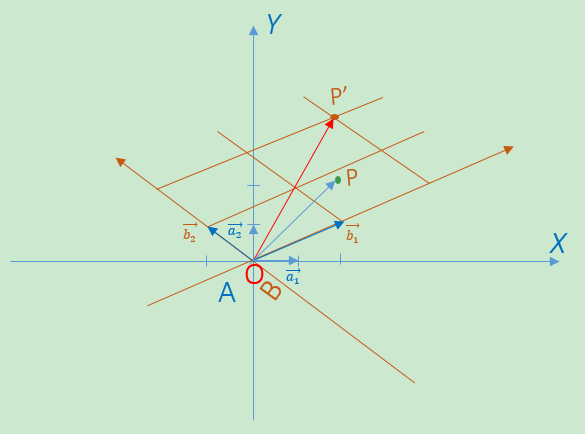站在不同的角度看同一个矩阵变换Bx⃗ $B\vec{x}$：

1).  位置变换：

A坐标系中：

向量OP→=$\vec{OP} = \begin{bmatrix}2\\2\end{bmatrix}$ ， 向量b1→=$\vec{b_1} = \begin{bmatrix}2\\1\end{bmatrix}$，b2→=[−11]$\vec{b_2}= \begin{bmatrix}-1\\1\end{bmatrix}$， 令矩阵B$B$ = [b1→b2→]$\begin{bmatrix}\vec{b_{1}}&\vec{b_{2}}\end{bmatrix}$，则矩阵变换Bx⃗ $B\vec{x}$会将OP→$\vec{OP}$变到OP′→$\vec{OP'}$。

2). 坐标系变换：

B坐标系中：

向量OP′→=$\vec{OP'} = \begin{bmatrix}2\\2\end{bmatrix}$

A坐标系中：

向量b1→=$\vec{b_1} = \begin{bmatrix}2\\1\end{bmatrix}$，b2→=[−11]$\vec{b_2}= \begin{bmatrix}-1\\1\end{bmatrix}$ ， 令矩阵B$B$ = [b1→b2→]$\begin{bmatrix}\vec{b_{1}}&\vec{b_{2}}\end{bmatrix}$，则矩阵变换Bx⃗ $B\vec{x}$会将OP′→$\vec{OP'}$从坐标系B变到坐标系A。

总结

于是，对任意坐标向量x⃗ $\vec{x}$，矩阵变换的组合M1M22M3...Mn$M_1M2_2M_3...M_n$ 作用于x⃗ $\vec{x}$存在两种解释。

x′→$\vec{x'}$=M1M22M3...Mn$M_1M2_2M_3...M_n$x⃗ $\vec{x}$的两种解释：

1). 坐标系不变，位置变换：

将M1M22M3...Mn$M_1M2_2M_3...M_n$看作从右到左依次作用的矩阵变换。首先Mn$M_n$变换， 将坐标向量x⃗ $\vec{x}$变换到了xn−1→$\vec{x_{n-1}}$=Mn$M_n$x⃗ $\vec{x}$，接着是Mn−1$M_{n-1}$变换，将坐标向量xn−1→$\vec{x_{n-1}}$变换到了xn−2→$\vec{x_{n-2}}$=Mn−1$M_{n-1}$xn−1→$\vec{x_{n-1}}$…。所有的中间结果都是同一个坐标系中的坐标向量，所有的变换都是针对同一个坐标系的变换。

2). 位置不变，坐标系变换：

将M1M22M3...Mn$M_1M2_2M_3...M_n$看作从左到右依次作用的矩阵变换。首先M1$M_1$变换，将M1坐标系中的坐标向量x1→$\vec{x_1}$转换为起始坐标系中的向量x′→$\vec{x'}$，x′→$\vec{x'}$=M1$M_1$x1→$\vec{x_1}$，然后是M2$M_2$变换，将M2坐标系中的坐标向量x2→$\vec{x_2}$转换成M1坐标系中的坐标向量x1→$\vec{x_1}$，x1→$\vec{x_1}$=M2$M_2$x2→$\vec{x_2}$…最后是Mn$M_n$变换，将Mn坐标系中的坐标向量x⃗ $\vec{x}$转换成Mn-1坐标系中的坐标向量xn−1→$\vec{x_{n-1}}$，xn−1→$\vec{x_{n-1}}$ =Mn$M_n$x⃗ $\vec{x}$ 。所有的中间结果都是不同坐标系中的坐标向量，且坐标向量的绝对位置没有改变（想象该点被一个钉子钉住）。从Mn坐标系的坐标向量x⃗ $\vec{x}$依次与MnMn−1...M3M2M1$M_nM{n-1}...M_3M_2M_1$矩阵相乘最终转换到起始坐标系对应的坐标向量x′→$\vec{x'}$的过程就是一系列前面提到过的追溯过程。
展开全文线性代数 OpenGL
• 对于以前数学学习不好的人来说，在机器人的坐标变换里，总是各种蒙。 这个图片来给你解答 机器人坐标系变换 坐标变换-旋转部分 二维坐标旋转的向量和几何表示
• ## 三维空间坐标系变换-旋转矩阵

万次阅读 多人点赞 2018-09-03 18:38:08
空间中三维坐标变换一般由三种... 这里以常见的世界坐标系与相机坐标系间的变换为例。 一、首先介绍从相机坐标系转换到世界坐标系，也就是比较通用的body到世界坐标系间的转换。  那么旋转的欧拉角按从世界坐标...
       空间中三维坐标变换一般由三种方式实现，第一种是旋转矩阵和旋转向量；第二种是欧拉角；第三种是四元数。这里先介绍旋转矩阵(旋转向量)与欧拉角实现三维空间坐标变换的方法以及两者之间的关系。

这里以常见的世界坐标系与相机坐标系间的变换为例。

一、首先介绍从相机坐标系转换到世界坐标系，也就是比较通用的body到世界坐标系间的转换。

那么旋转的欧拉角按从世界坐标系转换到相机坐标系的过程，先按z轴旋转、之后y轴旋转、之后x轴旋转，最终得到相机坐标系，得到的角度分别是yaw、pitch、roll，那么从相机坐标系到世界坐标系的旋转矩阵按如下方式定义: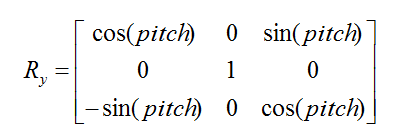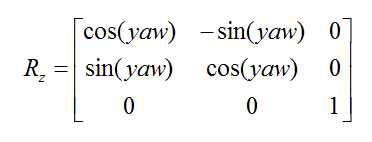那么得到的相机（body）坐标系到世界坐标系间的旋转矩阵为：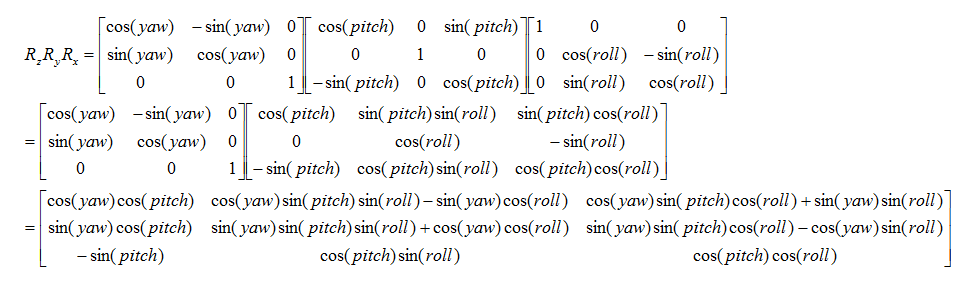cv::Mat IMUReader::angleToRotation(COORDINATES& carrier)
{
// R
cv::Mat R = cv::Mat::eye(3, 3, CV_32F);
R.at<float>(0, 0) = cos(carrier.yaw)*cos(carrier.pitch);
R.at<float>(0, 1) = cos(carrier.yaw)*sin(carrier.roll)*sin(carrier.pitch) - cos(carrier.roll)*sin(carrier.yaw);
R.at<float>(0, 2) = sin(carrier.yaw)*sin(carrier.roll) + cos(carrier.yaw)*cos(carrier.roll)*sin(carrier.pitch);
R.at<float>(1, 0) = cos(carrier.pitch)*sin(carrier.yaw);
R.at<float>(1, 1) = cos(carrier.yaw)*cos(carrier.roll) + sin(carrier.yaw)*sin(carrier.roll)*sin(carrier.pitch);
R.at<float>(1, 2) = cos(carrier.roll)*sin(carrier.yaw)*sin(carrier.pitch) - cos(carrier.yaw)*sin(carrier.roll);
R.at<float>(2, 0) = - sin(carrier.pitch);
R.at<float>(2, 1) = cos(carrier.pitch)*sin(carrier.roll);
R.at<float>(2, 2) = cos(carrier.roll)*cos(carrier.pitch);
return R;
}

对于平移矩阵为相机坐标系原点在世界坐标系下的坐标T，最终得到的坐标变换方程如下：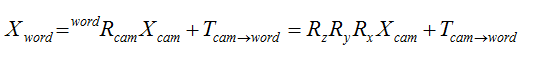其理解过程为从世界坐标系经过z，y，x的旋转以及平移T后得到相机坐标系，那么从相机坐标转换的世界坐标实际上是反变换过程，最后一步是x旋转，那么通过定义Rx为反旋转将相机坐标反旋转过来，之后依次是y，z，旋转后得到的值为与世界坐标系同方向，但是有一个平移的T的坐标系下的坐标，之后再加一个T坐标就得到了在世界坐标系下的坐标。

二、下面介绍从世界坐标系旋转到相机坐标系，这是一个正向旋转的过程，首先绕z，y，x旋转并平移T得到相机坐标系，那么从世界坐标系到与相机坐标系同向的坐标系是只经过旋转，那么旋转矩阵为：

RxRyRz，而此时三个维度的旋转分别是：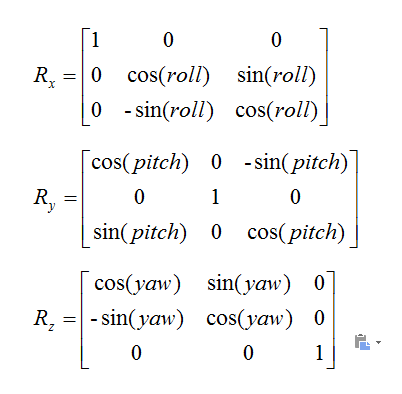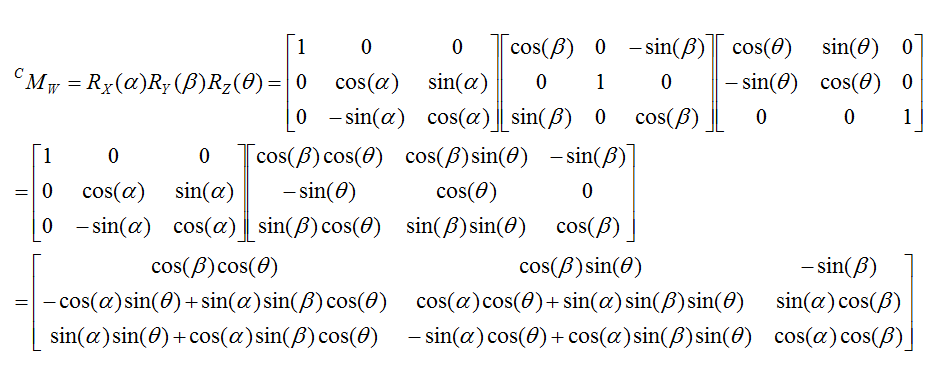对于平移矩阵是，此时坐标已经是与相机坐标系同向，但是与世界坐标系原点重合的坐标下的坐标，转换相机坐标系还需要加上的平移是世界坐标系原点在相机坐标系下的坐标T。

其理解过程就是一个在世界坐标系依照顺序进行z，y，x的旋转，之后再平移的过程。

其中对于z，y，x方向的旋转都是按照右手系定则，大拇指指向轴线方向，四指方向为旋转的正方向。

下面为之前整理，可能有错误，仅借鉴。

界坐标系下的坐标（Xw,Yw,Zw）转换到相机坐标系下（Xc，Yc,Zc）。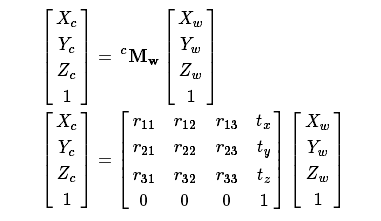那么这里涉及到旋转矩阵和平移矩阵。对于旋转我们有欧拉角描述，根据欧拉角计算出旋转矩阵。对于欧拉角指的是坐标系绕某个轴旋转的角度，那么从一个坐标系旋转到另一个坐标系需要三次旋转能够完成。对于绕坐标轴旋转分为两种，一种是绕原坐标系的固定轴旋转，一种是绕部分旋转后的坐标轴旋转，这里介绍比较常用的绕部分旋转后的坐标轴旋转。同时，对于绕坐标轴旋转其旋转的顺序也是有关系，如绕XYZ旋转和绕ZYX旋转。那么一种比较常用的表示方式是航空航天的偏航-俯仰-滚转表示法，其中坐标可以沿机头朝向为x轴，飞机左侧为y轴，飞机正上方为z轴。那么偏航为绕z轴旋转，之后俯仰是绕y轴旋转，滚转是绕x轴旋转，即该欧拉角的旋转顺序为ZYX.

上面介绍了旋转方式-欧拉角，下面介绍旋转矩阵的生成。这里分开不同轴讨论，先绕X轴旋转。我们定义旋转变换公式为：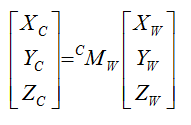其中，M为旋转矩阵，公式定义的是从世界坐标系向相机坐标系的转换，那么我们就按世界坐标向相机坐标旋转，如下：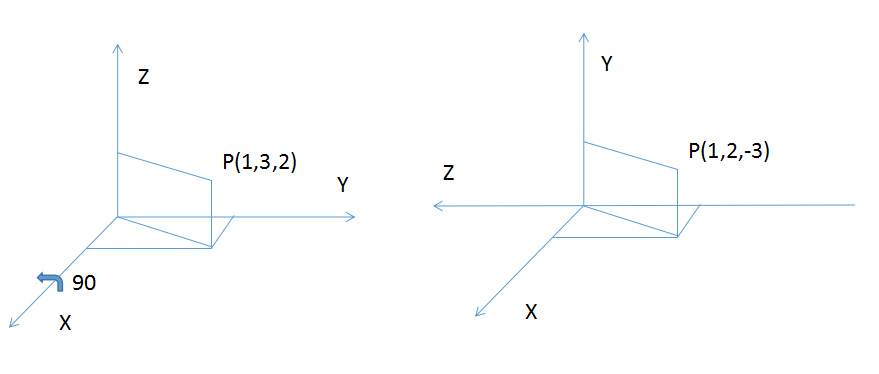这里我们定义了从左侧的世界坐标转到右侧的相机坐标，其旋转为绕X轴正向旋转90度，这里的正向旋转是以沿X轴方向看，顺时针旋转为正向，否则为负向。那么从世界坐标系向相机坐标系的旋转矩阵即为：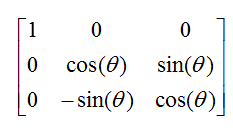此时从世界坐标向相机坐标的转换过程如下：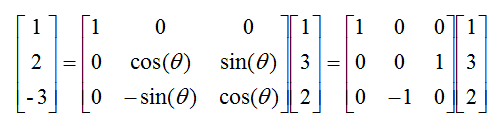所以，这里需要注意的是几点：（1）坐标是从世界坐标系向相机坐标系旋转，所有对于旋转方程是旋转矩阵乘以世界坐标得到相机坐标；（2）旋转是从世界坐标向相机坐标的旋转，即从源坐标系向目标坐标系旋转，这里是从世界坐标系向相机坐标系旋转；（3）旋转角度是沿着坐标轴的正向看，顺时针为正，逆时针为负；（4）旋转矩阵的形式如上，绕坐标轴旋转的轴坐标是不会变化的，所以是对应位置是1，接下来是0 cos(theta)  sin(theta), 在接下来是0   -sin(theta)  cos(theta)。

以上四个点的方向是与最终的变换公式相关的，有一个地方发生变化，那么最终的旋转变换公式也会发生变化，为了统一，那么这里按这样的规定，从而确定旋转变换公式的固定。

上面介绍了绕X轴旋转的情况，其它绕Y轴和Z轴的旋转矩阵相同，分别是：

绕Y轴旋转矩阵：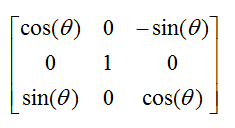绕Z轴旋转矩阵：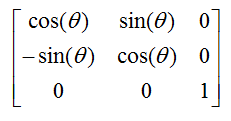那么假如从世界坐标系向相机坐标系的旋转过程中，显示绕Z轴旋转了theta，之后绕旋转后坐标系的Y轴旋转了beta，之后绕旋转后坐标系X轴旋转了alpha，那么最终的旋转矩阵如下：以上是对旋转矩阵的描述，那么对三维坐标系的转换，除了包含旋转，还要包含平移。即坐标系先从源旋转到与目标坐标系相同的方向，之后再平移。对于平移这里也规定从源坐标向目标坐标系。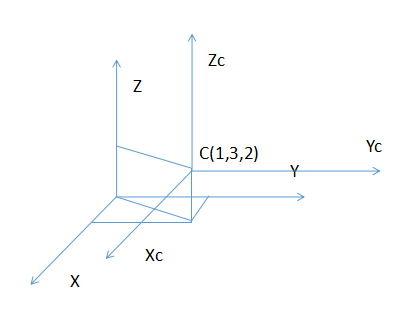这里目标坐标系在源坐标系下的坐标是(1,3,2),那么从源坐标系转到目标坐标系的变换即为：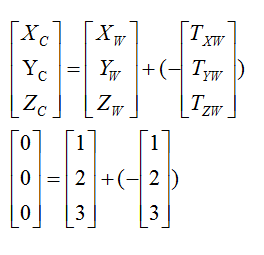于是这里规定几点：（1）变换是从源坐标系到目标坐标系，这里是从世界坐标系到相机坐标系；（2）平移变换是目标坐标系原点在源坐标系旋转到与目标坐标系相同方向后的坐标系下的坐标，这里即变换矩阵是目标坐标系原点在世界坐标系变换为与目标坐标系相同方向后在变换后世界坐标系中的坐标位置；（3）此时的变换矩阵需要加上负号。

如果不加负号，也可以将变换矩阵定义为源坐标系原点在目标坐标系下的位置，这里不用变换源坐标系。经过以上步骤可以将源坐标系中的坐标点转换到目标坐标系下。

对于旋转矩阵的旋转向量以及欧拉角的关系与变换：

对于旋转矩阵与旋转向量：

上面介绍了通过旋转矩阵和平移矩阵描述两个坐标系间的变换，其实也相当于表示了6自由度的三维刚体运行。但是旋转矩阵有几个缺点：SO(3)的旋转矩阵有9个量，但一次旋转只有3个自由度。因此，这种表达式冗余。而且对于旋转矩阵自身也有约束，它必须是正交矩阵，且行列式为1，这些约束会使求解变得困难。一种更紧凑的方式是使用旋转xian向量描述。即对于坐标系的旋转，任意旋转都可以用一个旋转轴和一个旋转角来刻画。于是，我们可以使用一个向量，其方向与旋转轴yizh一致，而长度等于旋转角。这种向量称为旋转向量(或轴角，Axis-Angle)。这种表示法只需一个三维向量即可描述旋转。对于xuan旋转向量和旋转矩阵间的转换可以通过罗德里格斯公司（Rodrigues Formula）来计算。

对于旋转矩阵与欧拉角：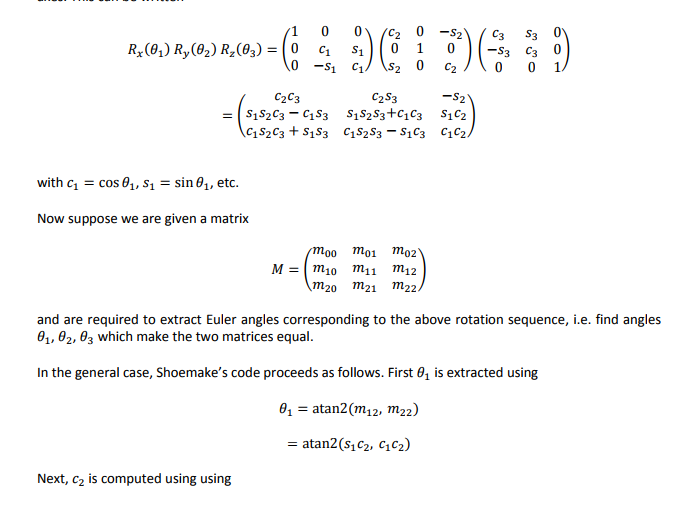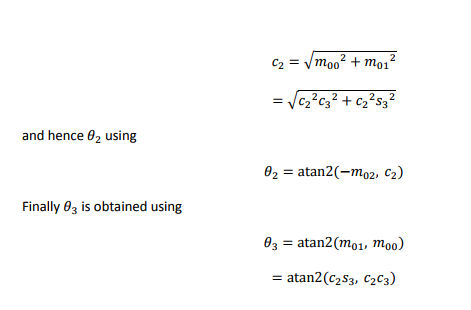展开全文• TF坐标系变换1. 概述2. 代码3. 最终结果4. 参考链接 1. 概述 使用代码实例来理解tf发布信息中的位姿变换具体含义：在父坐标系下描述父坐标系到子坐标系的变换关系，希望能帮助大家，同时给自己一个参考。 2. 代码 ...
TF坐标系变换1. 概述2. 代码3. 最终结果4. 参考链接
1. 概述
使用代码实例来理解tf发布信息中的位姿变换具体含义：在父坐标系下描述父坐标系到子坐标系的变换关系，希望能帮助大家，同时给自己一个参考。
2. 代码
参考代码如下
#include <ros/ros.h>
#include <tf/transform_broadcaster.h>

int main(int argc, char** argv){
ros::init(argc, argv, "my_tf_broadcaster");
ros::NodeHandle node;

tf::TransformBroadcaster br;
tf::Transform transform;

ros::Rate rate(10.0);
while (node.ok()){
//从父坐标系到子坐标系变换，是在父坐标系下的描述。
transform.setOrigin( tf::Vector3(0.0, 2.0, 0.0) );
transform.setRotation( tf::Quaternion(0, 0, 0, 1) );
br.sendTransform(tf::StampedTransform(transform, ros::Time::now(), "turtle1", "carrot1"));
rate.sleep();
}
return 0;
};

3. 最终结果
以turtle1坐标系为世界坐标系（固定坐标系）。从图中可以看到刚才发布的tf信息所表示的含义：

表示从父坐标系"turtle1"到子坐标系 "carrot1"的变换为[0.0, 2.0, 0.0]，（其实可以认为当前只有父坐标系，因此只能得到在父坐标系下的变换关系），这个变换关系是在父坐标系下的描述，如果是子坐标系的描述，那么变换关系会变成[0.0, -2.0, 0.0]。4. 参考链接
TF官方教程


展开全文ubuntu
• 欢迎关注公众号：编程外星人，加入技术交流群 无人机的状态估计包括姿态解算和速度位置估计，为了进行状态估计，我们首先... 我们来讨论坐标系变换的相关内容。假设在3维直角坐标系x^0、y^0、z^0中坐标系以z^0为转...欢迎关注公众号：编程外星人，加入技术交流群       无人机的状态估计包括姿态解算和速度位置估计，为了进行状态估计，我们首先需要了解状态估计所需要用到的4个坐标系。这4个坐标系为：传感器坐标系（sensor）、机体坐标系（body）、本地坐标第（local）、全局坐标系（global）。        我们来讨论坐标系变换的相关内容。假设在3维直角坐标系x^0、y^0、z^0中坐标系以z^0为转轴按逆时针旋转θ之后得到新的坐标系坐标系x^1、y^1、z^1，那么在原坐标系下的向量p在源坐标系下的坐标和新坐标系下的坐标如图所示：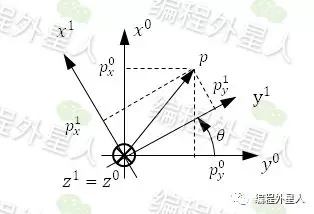于是有：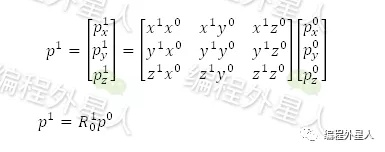其中，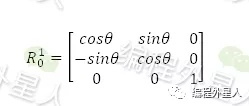其中，R_0^1为坐标系0变换到坐标系1的变换矩阵，也就是说，坐标系变化后，向量p在原坐标系下的坐标左乘变换矩阵得到其在变换后的坐标系下的坐标。变换矩阵是以z轴为转轴的，我们再给出分别以x轴和y轴为转轴的变换矩阵：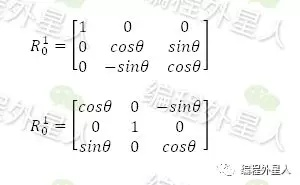设无人机的3个姿态角分别为：                ∅：滚转角（Roll）；                θ：俯仰角（Pitch）；                φ：航向角（Yaw）。        于是，当无人机产生滚转角、俯仰角和航向角之后，其变换矩阵为：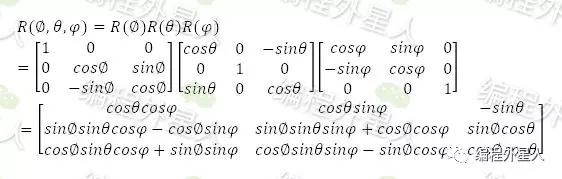注意，3个单坐标轴旋转的变换矩阵相乘的顺序不同，所得到变换矩阵的结果也是不同的。因此，在实际应用用通常采用四元数来进行坐标变换。由姿态角到四元数的转换公式如下：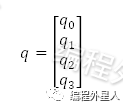其中，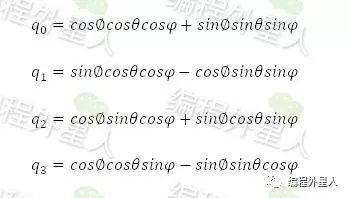四元数的变换矩阵为：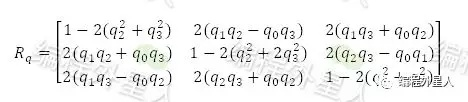接下来我们通过一个例子来简单说明变换矩阵的作用：设在无人机机体坐标系中得到的当前机体的速度v^b为：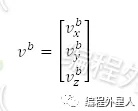当前姿态角为：∅、 θ、 φ，将姿态角转为四元数并得到其变换矩阵中的R_q，于是无人机在本地坐标系下的速度v^l为：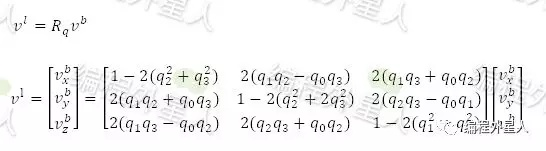坐标变换是无人机姿态解算以及速度和位置估计的重要工具，在数据融合时需要将各个传感器数据以及GPS所采集到的数据从自个的坐标系通过变换矩阵转换到本地坐标系当中，然后参与各项计算。欢迎关注公众号：编程外星人，加入技术交流群
展开全文• 本篇文章先定义坐标系变换，然后讲解什么是坐标系的对齐，最后说明怎么对一个物体做非刚体的对齐。 三维空间的点要像用数学来表示的话，就必须要要先建立坐标系。只有确定了坐标系，才能给每个3d的点一个坐标值。 ...
• 在SLAM中经常用到空间点的坐标变换。...开始的时候我有这样的疑问：T表示世界坐标到相机坐标系变换，而计算的是点坐标从相机坐标系到世界坐标系变换结果，为什么可以直接乘呢？而不是反向的旋转...
• 坐标系变换是图形学中最基础的部分，一个物体从建模到显示在屏幕，经历了从物体坐标系到世界坐标系，再从世界坐标系到观察坐标系，最后通过投影到2D平面，再变换到屏幕坐标等一系列过程。  投影矩阵  我们可以...
• 基于MATLAB的二维坐标系变换，陈兆林，张书毕，利用MATLAB的Delaunay函数功能将整个测区内的已知控制点连成三角网并计算各个三角形区域内的坐标转换参数，利用不同三角形区域内的转�
• ## 坐标系变换的理解

千次阅读 2018-10-01 11:40:25
在一个坐标系旋转和移动物体=把物体的点从自己的局部坐标系变换到世界坐标系=直接表示物体位置和朝向的矩阵 这里世界坐标系就是旋转前的坐标系，也是第一帧的坐标系 投影矩阵=把在世界坐标系下表示的物体的点变换...
• 坐标系变换通常包括：世界坐标系-物体坐标系变换、物体坐标系-世界坐标系变换和世界坐标系-屏幕坐标系变换（一个二维平面坐标系，即显示器平面，是非常标准的笛卡尔坐标系的第一象限区域）。 世界坐标系-物体坐标...
• 原文发布时间：2010-08-17作者：乱马 对于FME进行坐标系变换，涉及两种不同的变换，一个是基准面（Datum）发生变换，另一个基准面不发生变换。本文对第一种变换进行描述。 对于基准面发生变换的坐标系变换，在FME ...
• 原文地址：顶点法向量从物体坐标系变换到世界坐标系3维网格模型的顶点的法向量一开始是定义在模型坐标系中的，在将模型布置在场景中后，根据光照模型计算颜色时需要用到顶点法向量，由于光照都是在世界坐标系中进行...
• 本文档描述了将左手坐标系中的数据转换为右手坐标系中的数据的过程，包括顶点位置，平移，旋转变换等，右手坐标系转换为左手坐标系同样适用。
• ## 平面坐标系变换

千次阅读 2018-11-14 18:44:38
平面坐标系变换 flyfish x′=xcos⁡θ+ysin⁡θx&amp;#x27; = x \cos \theta + y \sin \theta x′=xcosθ+ysinθ y′=−xsin⁡θ+ycos⁡θy&amp;#x27; = - x \sin \theta + y \cos \theta y′=−xsinθ+ycos...
• SE2（李群二维坐标系变换） 坐标系间的坐标变换公式 坐标系示意图 说明： 为世界坐标系，为子坐标系，为坐标系中一点，点在系中的坐标为，在系中的坐标为，为子坐标系原点在世界坐标系中的坐标，为子坐标系与...
• 但是后来发现跟踪效果不好，去查验imu数据时，发现，我采集保存的imu数据格式没有和euroc数据集中的imu数据保存格式统一，所以需要研究的是在euroc用于数据采集的设备中的相机和imu之间的坐标系变换关系，我的设备...
• ## 3D坐标系变换

万次阅读 2011-03-30 08:38:00matrix 扩展 c
• 变换算子左乘：表示该变换是相对固定坐标系变换 变换算子右乘：表示该变换是相对动的坐标系（新坐标系）变换。 这一节里Jungle将在上一篇文章和变换算子的基础上，总结一下在机器人运动学分析里面，机器人关节坐标...
• [含R的最终表达式]三维空间坐标系变换-旋转矩阵 https://blog.csdn.net/fireflychh/article/details/82352710 [旋转顺序zxy]三维坐标旋转矩阵 https://blog.csdn.net/lz20120808/article/details/50809397 .....计算机视觉
• 所谓的坐标系变换就是调用某个函数后得到的坐标。 关系如下图：（当时小谢给我画的~） 绿色框框内的表示需要自己写代码的~     齐次坐标（Homogeneous coordinates）   三维顶点(x,y,z)，引入新分量w，...
• 1.坐标系变换：https://blog.csdn.net/waeceo/article/details/50580607
• Matlab Robotics Toolbox–坐标系变换 摘要 坐标变换一般可由旋转变换和平移变换两部分构成，上一篇介绍了Robotics Toolbox旋转变换的相关函数，这一篇介绍坐标系齐次变换的函数。 平移变换 （1）创建平移变换矩阵 ...matlab 机器人工具箱 toolbox...